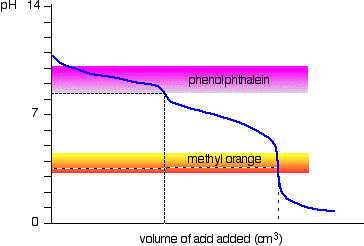# Can you help with this titration calculation ?

## A 1 litre solution contains a mixture of sodium hydroxide and sodium carbonate. 25 ml is removed and titrated against 0.1 M hydrochloric acid using phenolphthalein indicator. The mean titre recorded is 1.25 ml of 0.1 M HCl. The titration is repeated with methyl orange as indicator. The mean titre recorded is 2.35 ml of 0.1 M HCl. Calculate the mass of sodium hydroxide and sodium carbonate in the original solution.

Apr 11, 2017

$\textsf{{m}_{N a O H} = 0.024 \textcolor{w h i t e}{x} g}$

$\textsf{{m}_{N {a}_{2} C {O}_{3}} = 0.47 \textcolor{w h i t e}{x} g}$

#### Explanation:

We need to understand what happens when sodium carbonate is titrated with strong acid:

In the first part of the titration the carbonate ions are protonated:

$\textsf{C {O}_{3}^{2 -} + {H}^{+} \rightarrow H C {O}_{3}^{-}}$

The equivalence is at just over pH 7

At about pH 3 the 2nd protonation occurs:

$\textsf{H C {O}_{3}^{-} + {H}^{+} \rightarrow C {O}_{2} + {H}_{2} O}$

Effervescence due to the formation of $\textsf{C {O}_{2}}$ is observed.

The pH change during the titration is shown below:You can see that the choice of indicator is important.

Phenolphthalein will detect the first equivalence point, while methyl orange will detect the second equivalence point.

In this example we have a mixture of $\textsf{N a O H}$ and $\textsf{N {a}_{2} C {O}_{3}}$ solutions.

If phenolphthalein is used then the two reactions occurring in the flask are:

$\textsf{C {O}_{3}^{2 -} + {H}^{+} \rightarrow H C {O}_{3}^{-}}$

$\textsf{{H}^{+} + O {H}^{-} \rightarrow {H}_{2} O}$

$\textsf{{n}_{{H}^{+}} = c \times v = 1.25 \times \frac{0.1}{1000} = 1.25 \times {10}^{- 4}}$

$\therefore$$\textsf{{n}_{C {O}_{3}^{2 -}} + {n}_{O {H}^{-}} = 1.25 \times {10}^{- 4}}$

Now the titration is carried out using methyl orange indicator. This detects the second protonation:

$\textsf{H C {O}_{3}^{-} + {H}^{+} \rightarrow C {O}_{2} + {H}_{2} O}$

You can see that an extra 1.10 ml is needed to effect this end - point.

The extra moles of $\textsf{{H}^{+}}$ added is:

$\textsf{{n}_{{H}^{+}} = c \times v = 0.1 \times \frac{1.10}{1000} = 1.10 \times {10}^{- 4}}$

$\therefore$$\textsf{{n}_{H C {O}_{3}^{-}} = 1.10 \times {10}^{- 4}}$

Since 1 mole of $\textsf{C {O}_{3}^{2 -}}$ produces 1 mole of $\textsf{H C {O}_{3}^{-}}$ we can say that:

$\textsf{{n}_{C {O}_{3}^{2 -}} = 1.10 \times {10}^{- 4}}$

$\therefore$$\textsf{{n}_{N a O H} = 1.25 \times {10}^{- 4} - 1.10 \times {10}^{- 4} = 0.15 \times {10}^{- 4}}$

Converting moles to grams using $\textsf{m = n \times {M}_{r}}$ :

$\textsf{{m}_{N {a}_{2} C {O}_{3}} = 1.10 \times {10}^{- 4} \times 106.0 = 116.6 \times {10}^{- 4} \textcolor{w h i t e}{x} g}$

This is the mass in 25 ml of solution. The mass in 1L is:

$\textsf{\frac{116.6 \times {10}^{- 4}}{25} \times {10}^{3} = \textcolor{red}{0.466 \textcolor{w h i t e}{x} g}}$ of $\textsf{N {a}_{2} C {O}_{3}}$

Now for the mass of $\textsf{N a O H}$ :

$\textsf{{m}_{N a O H} = 0.15 \times {10}^{- 4} \times 39.997 = 6.00 \times {10}^{- 4} \textcolor{w h i t e}{x} g}$

This is the mass in 25 ml of solution. The mass in 1L is:

$\textsf{\frac{6.00 \times {10}^{- 4}}{25} \times {10}^{3} = \textcolor{red}{0.024 \textcolor{w h i t e}{x} g}}$ of $\textsf{N a O H}$